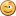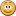## PlanetWerks performance tweaks

Apr 29, 2008

A great deal of work has gone into making the PlanetWerks widget run as smoothly as it possibly can. I just thought I'd share a few tweaks which have gone into the latest version (released later this week hopefully) and a few earlier versions.

Fully Matrix Driven
Up until version 2.1, the transformations performed on the orbit ellipses to orient them correctly in 3D space were done by three sets of discrete parametric equations. This simply means that once a planetoid was placed on the orbit ellipse, it was rotated once, then rotated again to incline it to the ecliptic, then rotated a third time to point the periapsis in the correct direction. That's three separate rotations before each planetoid can be displayed.

Using matrix multiplication, it is possible to combine all of these rotations into a single rotation; and since the orbit of each planetoid does not change within PlanetWerks (they do over time IRL), I can do all that preparation before the animation starts and then apply one rotation per animation frame.

Reducing Calculation Load
I spent a lot of time reviewing what actually gets executed during a single frame of animation, and trying to cut out any calculations which could be pre-calculated for speed, or algorithms which might be faster in a different form.

First of all, when I switched to the matrix driven rotation method, I had a nice function which performed the rotation from a matrix upon a vector (an [x, y, z] point on the ellipse):

```Math.matrixVector = function(m, v) {
for (var x = 0, output = []; x < m.length; x++) {
output[x] = 0;
for (var y = 0; y < m.length; y++)
output[x] += m[x][y] * v[y];
}
};```

This slick function would handle matrices and vectors of any size, but it also has a lot of loop overhead. Since I was only ever multiplying 3x3 matrices with 3-coordinate vectors, I unrolled all the loops and calculated each term manually.

```Math.matrixVector = function(m, v) {
return [
m * v + m * v + m * v,
m * v + m * v + m * v,
m * v + m * v + m * v
];
};```

This method turned out to be considerably faster, which is a good thing since this function is executed for every visible planetoid for every frame of animation in PlanetWerks!

Another thing I tried to remove was the creation of unnecessary variables. Creating a new variable using "var" can be handy, but it also entails a small overhead for both allocating new memory and garbage collection once it goes out of scope.

Since PlanetWerks 2.0, the user rotation of the system is driven by quaternions, which are simple 3 coordinate vectors with an added orientation coordinate that keeps them stable after many sequential rotations. Every time the user rotated the system, the rotation function would fire and update a global rotation matrix. When a key is held down, this rotation function can be called many times per second, so it is a good thing to optimise here. In version 2.1, the bottom section of this function looked like this:

```var angle = 2 * this.math.acos(this.quat), c = this.math.cos(angle), s = this.math.sin(angle), C = 1 - c;
var scale = this.math.sqrt(this.quat * this.quat + this.quat * this.quat + this.quat * this.quat);
var x = this.quat / scale, y = this.quat / scale, z = this.quat / scale;

var xs = x * s, ys = y * s, zs = z * s;
var xC = x * C, yC = y * C, zC = z * C;
var xyC = x * yC, yzC = y * zC, zxC = z * xC;

this.rota = [
x * xC + c, xyC - zs, zxC + ys,
xyC + zs, y * yC + c, yzC - xs,
zxC - ys, yzC + xs, z * zC + c
];
this.moved = true;```

Look at all those created variables! It was surely reducing the number of duplicated calculations, but it was also causing memory allocation overhead. I did some benchmarking and found that it executed moderately faster once unrolled and calculated manually:

```var angle = 2 * this.math.acos(this.quat), c = this.math.cos(angle), s = this.math.sin(angle), C = 1 - c;
var scale = this.math.sqrt(this.quat * this.quat + this.quat * this.quat + this.quat * this.quat);
var x = this.quat / scale, y = this.quat / scale, z = this.quat / scale;

this.rota = [
[x * x * C + c, x * y * C - z * s, z * x * C + y * s],
[x * y * C + z * s, y * y * C + c, y * z * C - x * s],
[z * x * C - y * s, y * z * C + x * s, z * z * C + c]
];```

More could probably be done here to reduce the number of new variables created, but readability is already getting strained to the limit.

Another thing I discovered is that cleverly combining assignments can slow execution speed by a very small amount, but still significantly. I benchmarked the following two groups of statements in Opera 9.5b2 and found that the latter, while not always the fastest, consistently executed about 5% faster than the former group:

Slower, compact, not very readable...

```this.appHeight2 = (this.math.round(tbx.ratio * (this.appWidth = this.math.max(1, this.appWidth)))) / 2 + 10;
this.appWidth2 = this.math.round(this.appWidth) / 2 + 10;
this.appAbsX = this.math.abs(this.appX = this.math.atan2(tbx.x / 2, tdtbxz) * this.factor);
this.appAbsY = this.math.abs(this.appY = this.math.atan2(tbx.y / 2, tdtbxz) * this.factor);
this.appPosX = this.math.round(this.appX + this.width2);
this.appPosY = this.math.round(this.appY + this.height2);```

Faster, longer, but also more readable...

```this.appWidth = this.math.max(1, this.appWidth);
this.appHeight = this.math.round(tbx.ratio * this.appWidth);
this.appWidth = this.math.round(this.appWidth);
this.appHeight2 = this.appHeight / 2 + 10;
this.appWidth2 = this.appWidth / 2 + 10;
this.appX = this.math.atan2(tbx.x / 2, tdtbxz) * this.factor;
this.appY = this.math.atan2(tbx.y / 2, tdtbxz) * this.factor;
this.appAbsX = this.math.abs(this.appX);
this.appAbsY = this.math.abs(this.appY);
this.appPosX = this.math.round(this.appX + this.width2);
this.appPosY = this.math.round(this.appY + this.height2);```

Binary Flags
Some functions in PlanetWerks called for systems of flags which directed the main animation function to perform in a certain way. Previously I was using a separate variable storing a Boolean for each flag. For example, there were separate flags set if the system had "moved", been "zoomed" or "rotated". In 2.2, these three flags have been combined into a single binary value with places set for each flag. 001 means the system has moved, 010 means the system has been zoomed, and 100 means the system has been rotated. Using Javascript's binary math & operator, it is easy to check whether one or multiple values have been set. If I want to know if the zoomed bit has been set, I simply "and" the set with 2:

`if (this.flags & 2) { /* system has been zoomed */ }`

Since 2 in binary is 010, the & operator means, if a bit is equal to 1 in both variables, set it in the result. So say the system had been rotated and zoomed: 110 & 010 = 010. And if the system had been rotated, moved, but not zoomed: 101 & 010 = 000. You can even get more complicated; suppose I wanted to know if the system had been zoomed or rotated. I would simply "and" the flag set with a combination of the zoomed and rotated flags, in other words: 011. Now if either the zoomed or rotated flag is set in the flag set, we will get a true result from the & operator.

I really don't know if this is faster than having separate variables for each, but having one entry in the lookup table instead of three has got to count for somethingThat's it for now. Hopefully I will get PlanetWerks 2.2 out there before I head off on holidays!⇐ Coming soon... PlanetWerks 2.2 :) Guys, I am telling you... Dragonland is the best band ever! Srsly! ⇒